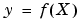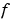MATLAB Function Referencegriddatan

Data gridding and hypersurface fitting (dimension >= 2)

Syntax

• ```yi = griddatan(X,y,xi)
yi = griddatan(`x,y,z,v,xi,yi,zi`,method)
```yi = griddatan(x,y,z,v,xi,yi,zi,method,options)
``````

Description

```yi = griddatan(X, y, xi) ``` fits a hyper-surface of the formto the data in the (usually) nonuniformly-spaced vectors (`X`, `y`). `griddatan` interpolates this hyper-surface at the points specified by `xi` to produce `yi`. `xi` can be nonuniform.

`X` is of dimension `m`-by-`n`, representing `m` points in n-dimensional space. `y` is of dimension `m`-by-`1`, representing `m` values of the hyper-surface(`X`). `xi` is a vector of size `p`-by-`n`, representing `p` points in the n-dimensional space whose surface value is to be fitted. `yi` is a vector of length `p` approximating the values(`xi`). The hypersurface always goes through the data points (`X`,`y`). `xi` is usually a uniform grid (as produced by `meshgrid`).

```yi = griddatan(x,y,z,v,xi,yi,zi,method) ``` defines the type of surface fit to the data, where `'method'` is one of:

 `'linear'` Tessellation-based linear interpolation (default) `'nearest'` Nearest neighbor interpolation

All the methods are based on a Delaunay tessellation of the data.

If `method` is `[]`, the default `'linear'` method is used.

`yi = griddatan(x,y,z,v,xi,yi,zi,method,options)` specifies a cell array of strings `options` to be used in Qhull via `delaunayn`.

If `options` is `[]`, the default options are used. If `options` is `{''}`, no options are used, not even the default.

Algorithm

The `griddatan` methods are based on a Delaunay triangulation of the data that uses Qhull . For information about Qhull, see http://www.qhull.org/. For copyright information, see http://www.qhull.org/COPYING.txt.

See Also

`delaunayn`, `griddata`, `griddata3`, `meshgrid`

Reference

  Barber, C. B., D.P. Dobkin, and H.T. Huhdanpaa, "The Quickhull Algorithm for Convex Hulls," ACM Transactions on Mathematical Software, Vol. 22, No. 4, Dec. 1996, p. 469-483. Available in HTML format at http://www.acm.org/pubs/citations/journals/toms/1996-22-4/p469-barber.

  National Science and Technology Research Center for Computation and Visualization of Geometric Structures (The Geometry Center), University of Minnesota. 1993.

© 1994-2005 The MathWorks, Inc.